## Equations for Uniform Acceleration

### Summary

Velocity-time graphs can be used to derive various different formulas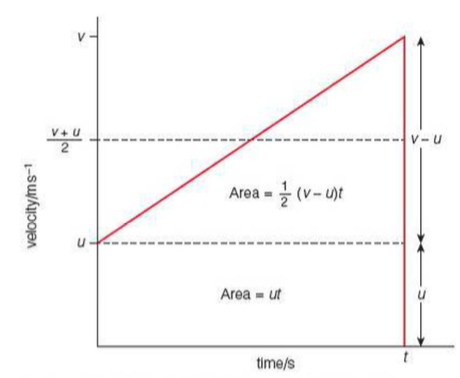This velocity-time graph shows a vehicle increasing its velocity with a consent acceleration, a, from an initial velocity, u, to a final velocity, v, over a time, t

The first equation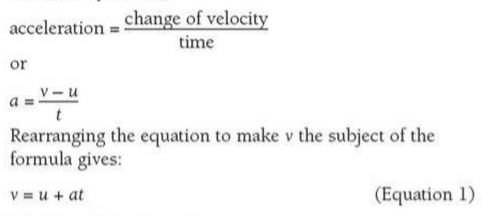The second equation

Displacement = average velocity x time

The average velocity is half way between the initial and final velocities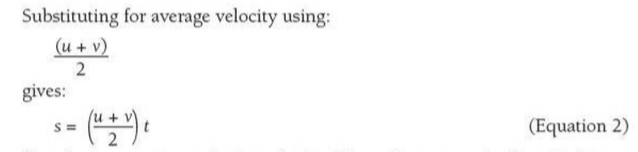The third equation

The displacement can also be calculated from the area under the velocity-time graph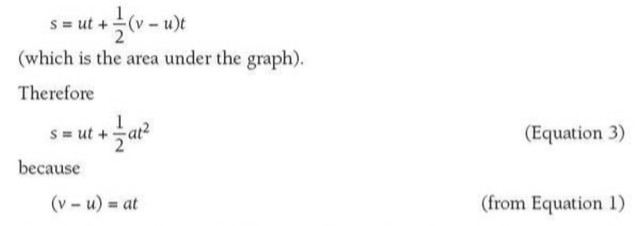The fourth equation

This links velocity, acceleration and displacement...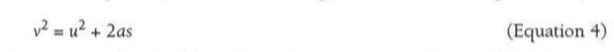There is no need to be able to derive these equations as they will be given in the exam. However, the derivation of the fourth equation is provided below: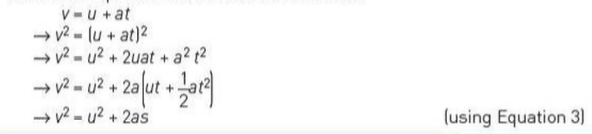### Example

Example 1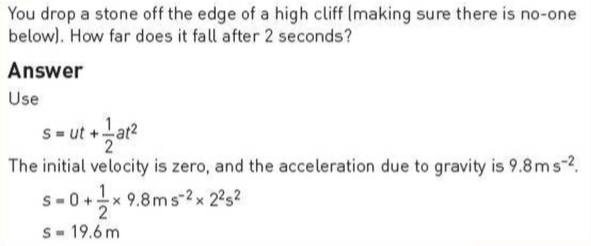Example 2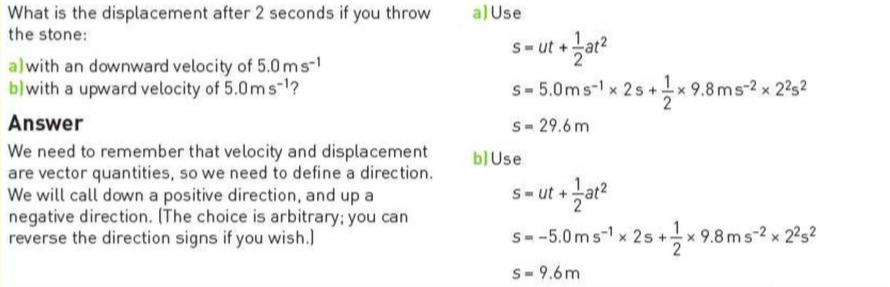Example 3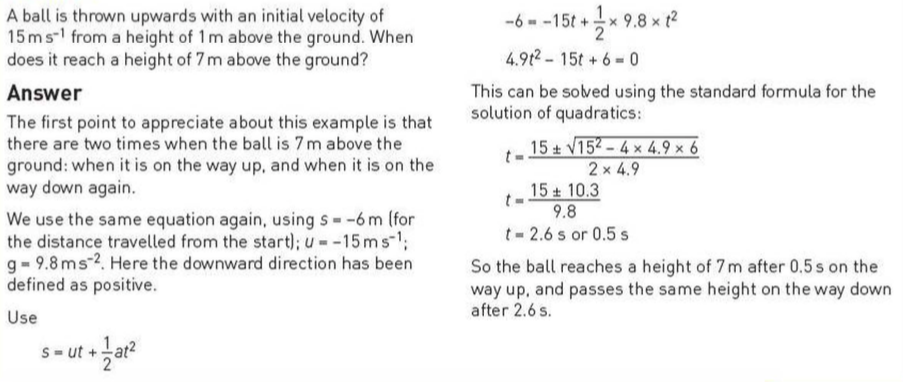### Acceleration due to gravity, g

Galileo Galilei demonstrated that gravity accelerates all masses at the same rate, provided air resistance is very small

Knowing this, you could calculate the acceleration due to gravity using the following: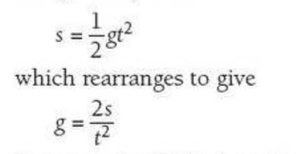For example, you could imagine Galileo dropping a ball from the Leaning Tower of Pisa (55m high) and taking 3.25s to fall: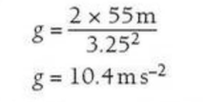### Terminal Speed

Terminal speed is the speed reached when the weight of an object in free fall is balanced by the drag forces (e.g. wind resistance) acting upwards

Drag is the name given to resistive forces experienced by an object moving through a fluid such as air or water

The size of the drag on a falling object increases with:

• Speed
• Surface area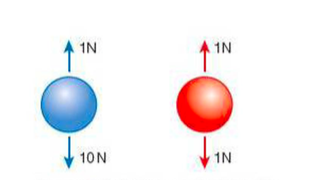This image shows how drag affects two similar balls - they are the same size/shape, but the blue ball weighs 10N and the red ball weighs 1N

Both balls fall at the same speed and both have an upward drag of 1N, but the blue ball continues to accelerate as it has a resultant downward force whereas the red ball moves at a constant speed because the force of gravity is balanced by the drag

• In other words, the red ball has reached its terminal speed

### Extra

Also see our notes on: